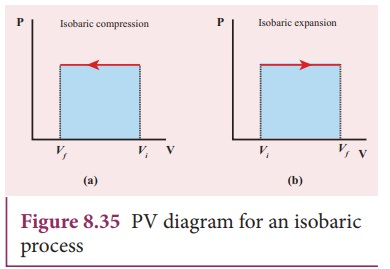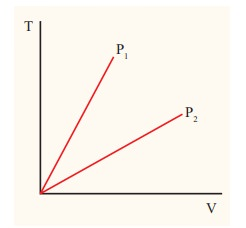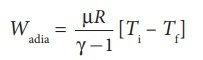Home | | Physics 11th std | Isobaric process

# Isobaric process

This is a thermodynamic process that occurs at constant pressure.

Isobaric process

This is a thermodynamic process that occurs at constant pressure. Even though pressure is constant in this process, temperature, volume and internal energy are not constant. From the ideal gas equation, we haveIn an isobaric process the temperature is directly proportional to volume.

V ŌłØ T          (Isobaric process) (8.44)This implies that for a isobaric process, the V-T graph is a straight line passing through the origin.

If a gas goes from a state (Vi ,Ti) to ( Vf ,Tf) at constant pressure, then the system satisfies the following equationExamples for Isobaric process:

(i) When the gas is heated and pushes the piston so that it exerts a force equivalent to atmospheric pressure plus the force due to gravity then this process is isobaric. This is shown in Figure 8.33(ii) Most of the cooking processes in our kitchen are isobaric processes. When the food is cooked in an open vessel, the pressure above the food is always at atmospheric pressure. This is shown in Figure 8.34The PV diagram for an isobaric process is a horizontal line parallel to volume axis as shown in Figure 8.35.

Figure 8.35 (a) represents isobaric process where volume decreases

Figure 8.35 (b) represents isobaric process where volume increasesThe work done in an isobaric process:

Work done by the gasIn an isobaric process, the pressure is constant, so P comes out of the integral,Where ŌłåV denotes change in the volume. If ŌłåV is negative, W is also negative. This implies that the work is done on the gas. If ŌłåV is positive, W is also positive, implying that work is done by the gas.

The equation (8.48) can also be rewritten using the ideal gas equation.

From ideal gas equationSubstituting this in equation (8.48) we getIn the PV diagram, area under the isobaric curve is equal to the work done in isobaric process. The shaded area in the following Figure8.36 is equal to the work done by the gas.The first law of thermodynamics for isobaric process is given byŌłåU = Q ŌĆō PDV

### EXAMPLE 8.19

The following graph shows a V-T graph for isobaric processes at two different pressures. Identify which one occurs at higher pressure.### Solution

From the ideal gas equation,

V = (┬ĄR/P)T

V-T graph is a straight line passing the origin.

The slope = ┬ĄR/P

The slope of V-T graph is inversely proportional to the pressure. If the slope is greater, lower is the pressure.

Here P1 has larger slope than P2. So P2 > P1.

Suppose the graph is drawn between T and V (Temperature along the x-axis and Volume along the y-axis) then will we still have P2 > P1?

### EXAMPLE 8.20

One mole of an ideal gas initially kept in a cylinder at pressure 1 MPa and temperature 27┬░C is made to expand until its volume is doubled.

(a)ŌĆé How much work is done if the expansion is (i) adiabatic (ii) isobaric (iii) isothermal?

(b) Identify the processes in which change in internal energy is least and is maximum.

(c) Show each process on a PV diagram.

(d) Name the processes in which the heat

(Take ╬│ = 5/3 and R=8.3 J mol-1 K-1)

### Solution

(a)  (i)  In an adiabatic process the work done by the system isTo find the final temperature Tf, we can use adiabatic equation of state.(ii)  In an isobaric process the work done by the system

W = P ŌłåV = P(Vf ŌĆō Vi)

and Vf = 2Vi         so W = 2PVi

To find Vi, we can use the ideal gas law for initial state. PiVi = RTiThe work done during isobaric process,

W = 2 ├Ś 106 ├Ś 24.9 ├Ś 10ŌłÆ4 = 4.9 kJ

(iii) In an isothermal process the work done by the system,In an isothermal process the initial room temperature is constant.

W = 1 ├Ś 8.3 ├Ś 300 ├Ś ln(2) = 1.7kJ

(b)  Comparing all three processes, we see that the work done in the isobaric process is the greatest, and work done in the adiabatic process is the least.(c)  The PV diagram is shown in the Figure.The area under the curve AB = Work done during the isobaric process

The area under the curve AC = Work done during the isothermal process

The area under the curve AD= Work done during the adiabatic process

From the PV diagram the area under the curve AB is more, implying that the work done in isobaric process is highest and work done in adiabatic process is least.

(d)  In an adiabatic process no heat enters into the system or leaves from the system. In an isobaric process the work done is more so heat supplied should be more compared to an isothermal process.

Tags : Thermodynamics , 11th Physics : UNIT 8 : Heat and Thermodynamics
Study Material, Lecturing Notes, Assignment, Reference, Wiki description explanation, brief detail
11th Physics : UNIT 8 : Heat and Thermodynamics : Isobaric process | Thermodynamics